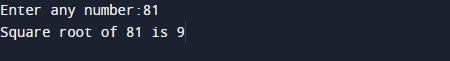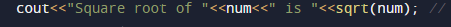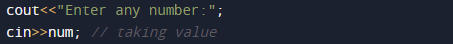# C++ Programs to Find Square Root of Number

In this tutorial you will learn about the C++ Programs to Find Square Root of Number and its application with practical example.

In this tutorial, we will learn to create a C++ program that will find Square Root of given number  using C++ programming.

## Prerequisites

Before starting with this tutorial we assume that you are best aware of the following C programming topics:

• Operators.
• Basic input/output.
• In-built functions.
• mathematical functions.

## What Is Square root?

Squares is found when numbers multiplying by itself Twice 5 * 5= 25. Where a square root of a number on getting multiplied by itself gives the original √25 = 5.
A square root of a number a is a number b such that =a.

## C++ Programs to Find Square Root of Number.

Firstly we declare required header file and variable and also initiates required values in variable. Here in this program we are going find Square root of a given number first of all we take a value from user and pass this value to in-built function to find Square root of a number.

## OutputFunction sqrt() takes a single  argument(num) and returns the square  root.In the above program, we have first declared and initialized a set variables required in the program.

• num = it will hold  given number.

with the help of sqrt() in-built function we can find the square root of given number of user.

Step by step explanation of this program.

1:First we declare  variable num.
2 :Then  take a value from user and assign to num.3 : Pass no variable to sqrt_root function (sqrt(num)).
4 : This function will find the square of given number .
5 : Print the value of sqrt(num).## Explanation of Square root.

So square root of a number is getting by multiplied itself gives the original as we can see in here √36 =6 .
so square root of 36 is 6. square root are written always in radical symbol (√).

In this tutorial we have learn about the C++ Programs to Find Square Root of Number and its application with practical example. I hope you will like this tutorial.